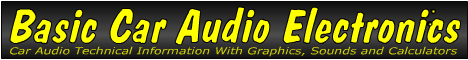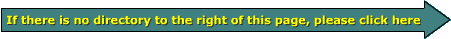Email   Home Pagex

 Web www.bcae1.com www.bcot1.com
Use F11 to go to full screen
Translate.

OhmAs was previously stated, the ohm is the unit of measure of a device's opposition to the flow of current. One ohm of resistance will allow only one amp of current to flow through a circuit when one volt of electrical force is applied to the circuit. If I am redundant in some of the definitions, it is to help to make things clearer.

All power sources are designed to operate into some sort of a load. Power supplies are generally designed to maintain a given voltage into some given load.

Let's say we have a 12 volt power supply that's designed to safely/reliably deliver a maximum of 1 amp of current. If we connect a load that has a resistance of 24 ohms, this will allow 1/2 of the power supply's max current to flow through the load.

If we connect a second 24 ohm load across the power supply's output terminals, the power supply will be delivering the maximum safe current output possible without damaging the supply.

If we connect a third load across the supply, the output current will be 1.5 times as much as the supply can safely deliver (assuming that the supply can maintain it's rated voltage under the heavy load) and the supply will likely blow a fuse or fail. The same thing happens when you connect too low of an ohm load (too many speakers) to an amplifier.

Lower ohm loads can allow the amplifier to produce more output current (which results in more output power - to be discussed later) but too low of an ohm load will cause the amplifier to fail. The amplifier expects to 'see' a certain minimum resistance (ohms) to assure a limited maximum current flow at maximum output.

Since this is a site is supposed to explain car audio... Lets say we have a 100 watt (we'll cover 'watts' soon) amplifier and it can drive a minimum ohm load of 4 ohms. This means that it can produce 100 watts into a 4 ohm load and any lower ohm load will cause the amplifier to fail. To produce 100 watts, the amplifier will have to deliver 5 amps of current. To produce a current flow of 5 amps into a 4 ohm load, it will have to develop 20 volts across the load (the voltage at the speaker terminals at full power will be 20 volts). Don't let all of these numbers confuse you, I'll cover all of this extensively later in the site. Now, the reason for the numbers... If you enter 4 ohms and 20 volts in the calculator below, you'll see that the current flow is 5 amps. If you reduce the ohm load to 2 ohms, the current flow will double. Since the max safe current output is only 5 amps and the lower ohm load causes more than 5 amps to flow, the amplifier may well be damaged by the lower ohm load.

• Use this program to calculate the current flow through a resistor.
• This calculator will show you how the voltage applied to a resistor and the resistor's value determine the current flow through the resistor.
• You may manually enter the the voltage and resistance.
• Notice how current flow INCREASES as the voltage increases and resistance decreases. You should also notice how the current flow DECREASES as the voltage decreases and resistance increases.
 Input ohms volts Output Current = Amps

 Note: When buying speakers, you must know what ohm load your amplifier needs to get full power from it. When you know that, you can then get the right speakers (either single voice coil or dual voice coil). I'll cover more on that in later chapters.

 You should remember: 1.The ohm is the unit of measure of a devices opposition to the flow of electrical current.

```

```calculator